# Sections of a Cone

#### notes

Let l be a fixed vertical line and m be another line intersecting it at a fixed point V and inclined to it at an angle α Fig.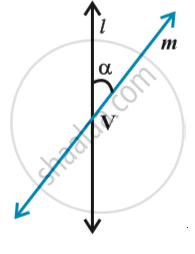Suppose we rotate the line m around the line l in such a way that the angle α remains constant. Then the surface generated is a double-napped right circular hollow cone  and extending indefinitely far in both directions Fig.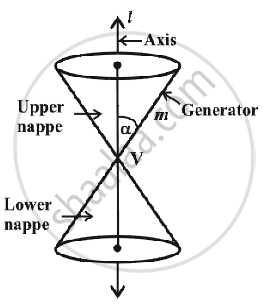the point V is called the vertex; the line l is the axis of the cone. The rotating line m is called a generator of the cone.  The vertex separates the cone into two parts called nappes.
If we take the intersection of a plane with a cone, the section so obtained is called a conic section. Thus, conic sections are the curves obtained by intersecting a right circular cone by a plane.
We obtain different kinds of conic sections depending on the position of the intersecting plane with respect to the cone and by the angle made by it with the vertical axis of the cone.  Let β be the angle made by the intersecting plane with the vertical axis of the cone Fig.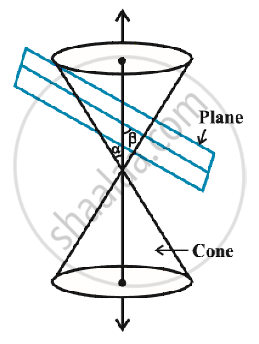1) Circle, ellipse, parabola and hyperbola:
When the planes cuts the nappe (other than vertex) of the cone, we have the following situations:
1)  When β = 90o,  the section is a circle Fig.
2)  When α < β <  90o, the section is an ellipse Fig.
3)  When β = α; the section is a parabola Fig.
(In each of the above three situations, the plane cuts entirely across one nappe of the cone).
4)  When 0 ≤ β < α; the plane cuts through both the nappes and the curves of intersection is a hyperbola Fig.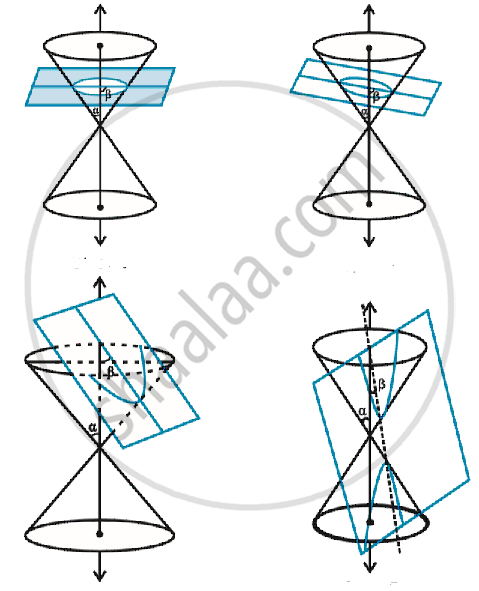2)  Degenerated conic sections:
When the plane cuts at the vertex of the cone, we have  the following  different cases:
1)  When α < β≤ 90^0, then the section is a point Fig.
2)  When β = α, the plane contains a generator of the cone and the section is a straight line Fig. It is the degenerated case of a parabola.
3) When 0 ≤β < α, the section is a pair of intersecting straight lines Fig.  It is the degenerated case of a hyperbola.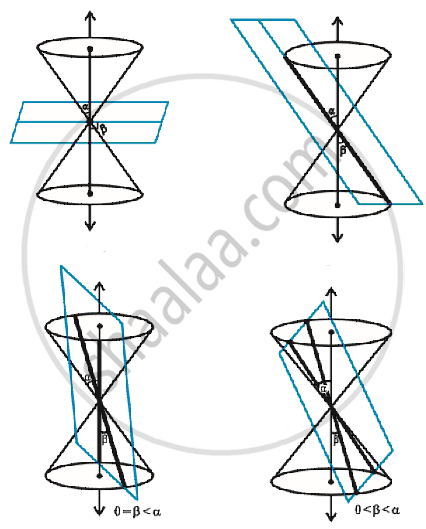If you would like to contribute notes or other learning material, please submit them using the button below.

### Shaalaa.com

Sections of a cone 1 [00:04:02]
S
0%